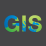# How to Automate "Calculate Geometry" Degrees Minutes Seconds within ModelBuilder?

1659
4
05-08-2019 06:02 AMNew Contributor III

Does anyone know how I can add the functionality of "Calculate Geometry" (Units: Degrees Minutes Seconds (DDD MM' SS.sss" [W|E]) and Degrees Minutes Seconds (DDD MM' SS.sss" [N|S])) within ModelBuilder? I figured out how to automate the calculation of both decimal degrees and State Plane coordinates using the "Calculate Field" tool (see below):

Decimal Degrees (using Python 9.3):

arcpy.PointGeometry(!Shape!.firstPoint,!Shape!.spatialReference).projectAs(arcpy.SpatialReference(4326)).firstPoint.X

arcpy.PointGeometry(!Shape!.firstPoint,!Shape!.spatialReference).projectAs(arcpy.SpatialReference(4326)).firstPoint.Y

State Plane Coordinates (using Python 9.3):

!SHAPE!.firstPoint.X

!SHAPE!.firstPoint.Y

Tags (4)
1 Solution

Accepted SolutionsbyMVP Legendary Contributor

You could try a code block in the field calculator.

``````def dd_mm_ss(dd, cal_long=True, use_sign=True, use_quad=True):
"""decimal degrees to deg dec min"""
deg_sign = u'\N{DEGREE SIGN}'
deg = int(dd)
if deg < 0:
deg = abs(deg)
else:
if not use_sign:
deg_sign = ""
mins, secs = divmod(dd*3600, 60)
degs, mins = divmod(mins, 60)
frmt = "{}{} {:0.0f}' {:05.2f}{}''".format(deg, deg_sign, mins, secs, quad)
return frmt

# expression box... python parser

dd_mm_ss(!YourField!, True, True, True)‍‍‍‍‍‍‍‍‍‍‍‍‍‍‍‍‍‍‍‍‍‍‍‍‍‍‍‍‍‍‍‍‍‍‍‍‍‍‍‍‍‍``````

yielding

``````# substituted a number for a field value to demo

dd_mm_ss(-75.5, True, True, True)

"75° 30' 00.00W''"‍‍‍‍‍``````

Of course, there are variants and you can mess with the code to suit your needs

4 RepliesbyMVP Legendary Contributor

You could try a code block in the field calculator.

``````def dd_mm_ss(dd, cal_long=True, use_sign=True, use_quad=True):
"""decimal degrees to deg dec min"""
deg_sign = u'\N{DEGREE SIGN}'
deg = int(dd)
if deg < 0:
deg = abs(deg)
else:
if not use_sign:
deg_sign = ""
mins, secs = divmod(dd*3600, 60)
degs, mins = divmod(mins, 60)
frmt = "{}{} {:0.0f}' {:05.2f}{}''".format(deg, deg_sign, mins, secs, quad)
return frmt

# expression box... python parser

dd_mm_ss(!YourField!, True, True, True)‍‍‍‍‍‍‍‍‍‍‍‍‍‍‍‍‍‍‍‍‍‍‍‍‍‍‍‍‍‍‍‍‍‍‍‍‍‍‍‍‍‍``````

yielding

``````# substituted a number for a field value to demo

dd_mm_ss(-75.5, True, True, True)

"75° 30' 00.00W''"‍‍‍‍‍``````

Of course, there are variants and you can mess with the code to suit your needsNew Contributor III

Thank you Dan. I tried running the above code and received the below error:

Start Time: Thu May 09 10:02:32 2019
ERROR 000539: Error running expression: dd_mm_ss(-110.7623741472387, True, True, True)
Traceback (most recent call last):
File "<expression>", line 1, in <module>
File "<string>", line 16, in dd_mm_ss
UnicodeEncodeError: 'ascii' codec can't encode character u'\xb0' in position 0: ordinal not in range(128)

Failed to execute (Calculate Field).

I'm using this code within ArcMap, not ArcPro - not sure if that has anything to do with Python 2 vs. Python 3.byMVP Legendary Contributor

big difference... python 2 doesn't support Unicode without some effort

try changing

deg_sign = u'\N{DEGREE SIGN}'

to

deg_sign = "d" or just ""

and

``````frmt = "{}{} {:0.0f}m {:05.2f}{}s".format(deg, deg_sign, mins, secs, "")

print(frmt)

-75d 30m 30.00s‍‍‍‍‍``````Occasional Contributor III

An alternative solution would be the Add Geometry Attributes geoprocessing tool.